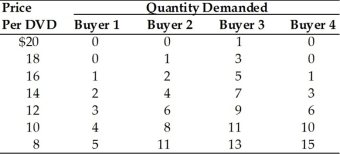• FAQ
• Contact/ Homework Answers / Economics / 101) An indirect or inverse relationship between price and quantity
Not my Question
Flag Content

# Question : 101) An indirect or inverse relationship between price and quantity

101) An indirect or inverse relationship between price and quantity demanded is

A) the market clearing price.

B) a change in demand.

C) a supply curve.

D) a demand curve.

102) By summing the quantities demanded by individuals at each price we obtain the

A) equilibrium price.

B) market demand curve.

C) market supply curve.

D) individual demand curve.

103) We are given the individual demand curves for all of the people that consume Good Y. Which statement is TRUE about the market demand curve for Good Y?

A) The market demand curve is obtained by horizontally summing the individual demand curves.

B) The market demand curve is obtained by vertically summing the individual demand curves.

C) The market demand curve cannot be obtained because information on prices is missing.

D) The market demand curve cannot be obtained from individual demand curves.

104) A market demand curve is found by

A) adding the prices each consumer would pay for each quantity.

B) adding the prices and the quantities demanded by a consumer.

C) adding the quantities demanded for each individual consumer at each price.

D) taking the demand curve of the "representative" consumer.105) Refer to the above table. What is the market quantity demanded of DVDs at a price of \$12?

A) 6

B) 9

C) 12

D) 24

106) Refer to the above table. What is the market quantity demanded at a price of \$8?

A) 5

B) 15

C) 35

D) 44

107) Refer to the above table. Suppose Buyer 2 leaves the market. What is the new market quantity of DVDs demanded at a price of \$10?

A) 33

B) 25

C) 22

D) 8

108) Demand is a schedule that shows

A) a set of possible prices for a good and the quantities of the good that will be purchased at each of those prices.

B) how much income it takes to afford various quantities of a good.

C) the relationship between the cost of producing a good and the price that sellers will charge.

D) how population changes will affect the amount of a good that is needed.

109) State the law of demand and illustrate it. Explain what is meant by the term "price" in the law of demand.

## Solution 5 (1 Ratings )

Solved
Economics 8 Months Ago 14 Views